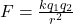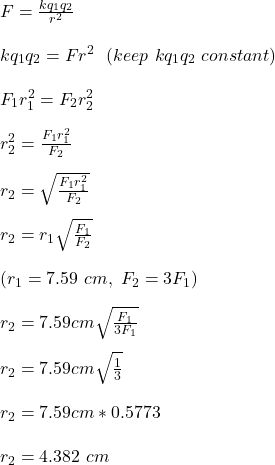Two small charged spheres are 7.59 cmcm apart. They are moved, and the force on each of them is found to have been tripled. How far apart ar

Question

Two small charged spheres are 7.59 cmcm apart. They are moved, and the force on each of them is found to have been tripled. How far apart are they now?

in progress 0
5 months 2021-08-16T05:10:59+00:00 1 Answers 0 views 0

The two small charged spheres are now 4.382 cm apart

Explanation:

Given;

distance between the two small charged sphere, r = 7.59 cm

The force on each of the charged sphere can be calculated by applying Coulomb’s law;where;

F is the force on each sphere

q₁ and q₂ are the charges of the spheres

r is the distance between the spheresTherefore, the two small charged spheres are now 4.382 cm apart.